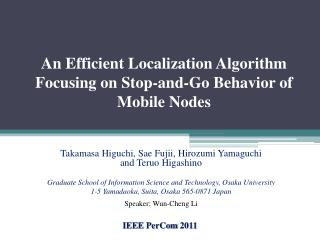DownloadDownload PresentationAn Efficient Localization Algorithm Focusing on Stop-and-Go Behavior of Mobile Nodes

# An Efficient Localization Algorithm Focusing on Stop-and-Go Behavior of Mobile Nodes

Télécharger la présentation## An Efficient Localization Algorithm Focusing on Stop-and-Go Behavior of Mobile Nodes

- - - - - - - - - - - - - - - - - - - - - - - - - - - E N D - - - - - - - - - - - - - - - - - - - - - - - - - - -
##### Presentation Transcript

1. An Efficient Localization Algorithm Focusing on Stop-and-Go Behavior of Mobile Nodes Takamasa Higuchi, SaeFujii, Hirozumi Yamaguchi and TeruoHigashino Graduate School of Information Science and Technology, Osaka University 1-5 Yamadaoka, Suita, Osaka 565-0871 Japan Speaker: Wun-Cheng Li • IEEE PerCom2011

2. Outline • Introduction • Network Model • State Decision Process • Localization Interval • Protocol Design • Simulation • Conclusion

3. Introduction • Location-aware services on cell phones have spread rapidly. • Car navigation systems • Pedestrian navigation applications

4. Introduction • However, to provide real-time position information to people indoor is still a big challenge. • Exhibition patrons • Museum visitors • Customers at shopping malls

5. Introduction • Rely on large amounts of fixed infrastructure for positioning also requires a lot of installation and maintenance costs

6. Motivation • Not all applications require accurate location information. • Allow a certain range of localization error

7. Problem • To accomplish acceptable accuracy of mobile nodes, frequency of position updates should be sufficiently high. • How a certain error range enables mobile nodes to locate and reduce excessive localization frequency reduction.

8. Goals • Propose an efficient localization algorithm of mobile nodes to • decrease the localization overhead • satisfy the constraint of tolerable position error of each sensor

9. Network Model AnchorNodes Unknown state Nodes Static state Nodes Moving state Nodes

10. Network Model • Each mobile node is assumed to have both an ultrasound ranging device and a wireless device • Applies a Time Difference of Arrival (TDoA) technique to measure the distance RF signals 10s 5m, (x1 , y1) A0 A1 (x1, y1) 20s ultrasound signals

11. Network Model • Each node Aiholds position (xi, y𝑖) and speed v𝑖 A2(x2, y2) v2= 0.0 m/s A1 (x1, y1) v1= 0.0 m/s measured distance d2 d1 d3 movement A3 (x3, y3) v3= 0.0 m/s A0 A0 (x0, y0) v0= 1.1 m/s d5 A5(x5, y5) v5= 0.0 m/s A4(x4, y4) v4= 1.0 m/s

12. State Decision Process • Measured distance from Aito a neighbor Ajis denoted as dj, and the estimated position of Ajas= (xj, yj). Aj(xj, yj) dj Ai

13. State Decision Process • represents the set of possible locations. A1 (x1 , y1) A4 (x4 , y4) d1 d4 d3 A3 (x3 , y3) A0 movement d3 d2 A3 (x’3 , y’3) A2(x2 , y2) Likelihood 1 Likelihood 2 Likelihood 3

14. Localization Interval • Speed viof Aiis estimated by the following formula. • 𝐼𝑣(𝑣𝑖) is updated in each localization process by the following function.

15. Localization Interval • The failure of movement detection by a single neighbor can be soon recovered by other neighbors. A0 A2 movement d1 d2 A0 d’2 d1 d’1 A1 A0 (x0, y0) v0= 0.0 m/s movement d’1 A1 A0 (x0, y0) v0= 0.0 m/s

16. Protocol Design • When a node performs localization, it broadcasts a Request To Measure (RTM) message before sending TDoAmeasurement signals. • The Network Allocation Vector (NAV)is used to determines the maximum transmission time delay. • is determined such that a node which has been delayed for longer time can have shorter backoff time using the following formula.

17. Simulation • QualNet

18. Simulation • Localization Error

19. Simulation • Tracking Error

20. Simulation • Localization Intervals

21. Simulation • Impact of Ranging Error Ranging Error[m]

22. Conclusions • This paper proposed a distributed cooperative algorithm to localize mobile nodes with a small number of anchor nodes. • Automatically adjusts localization frequency according to the estimated speed of nodes to reduce unnecessary localization attempts.

23. Thank you!